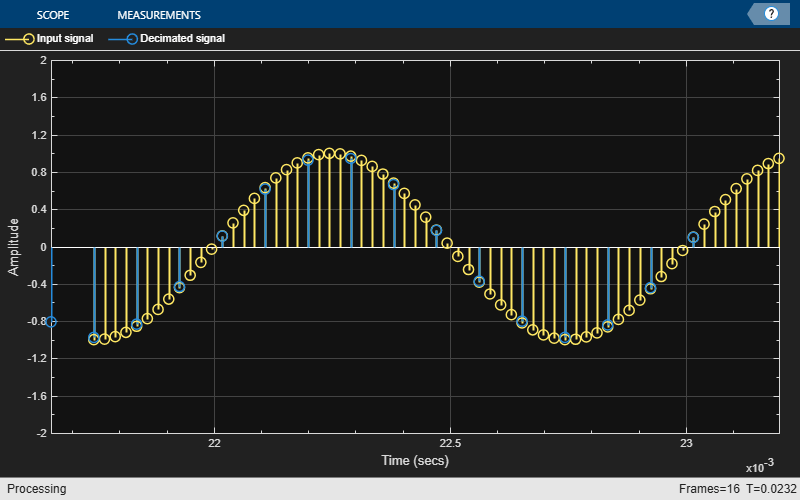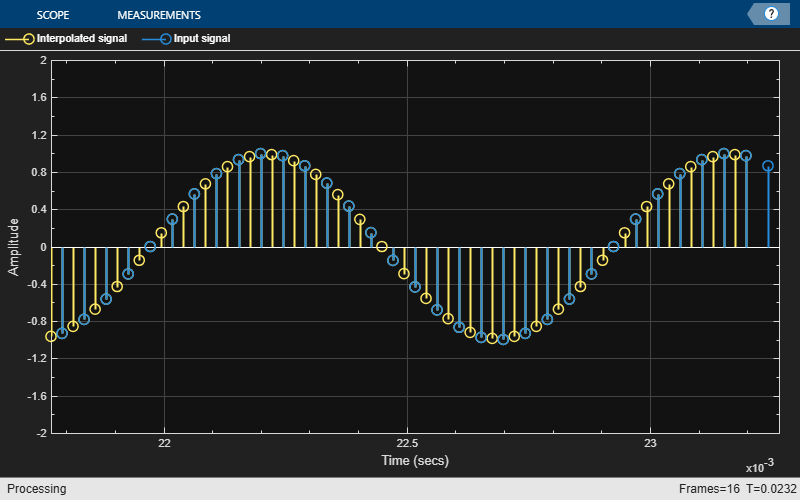Documentation

# info

Information about filter System object

## Syntax

``info(sysobj)``
``info(sysobj,infoType)``
``s = info(sysobj)``

## Description

example

````info(sysobj)` returns very basic information about the filter System object™, `sysobj`. The particulars depend on the filter type and structure.```

example

````info(sysobj,infoType)` returns the amount of filter information as specified by the `infoType`.```

example

````s = info(sysobj)` returns filter information in the variable `s`. You can also provide the optional `infoType` argument with this syntax.For more input options, see `info`.```

## Examples

collapse all

Obtain short-format and long-format information about a filter.

```d = fdesign.lowpass; f = design(d,'SystemObject',true); info(f)```
```ans = 6x35 char array 'Discrete-Time FIR Filter (real) ' '------------------------------- ' 'Filter Structure : Direct-Form FIR' 'Filter Length : 43 ' 'Stable : Yes ' 'Linear Phase : Yes (Type 1) ' ```
`info(f,'long')`
```ans = 45x45 char array 'Discrete-Time FIR Filter (real) ' '------------------------------- ' 'Filter Structure : Direct-Form FIR ' 'Filter Length : 43 ' 'Stable : Yes ' 'Linear Phase : Yes (Type 1) ' ' ' 'Design Method Information ' 'Design Algorithm : equiripple ' ' ' 'Design Options ' 'Density Factor : 16 ' 'Maximum Phase : false ' 'Minimum Order : any ' 'Minimum Phase : false ' 'Stopband Decay : 0 ' 'Stopband Shape : flat ' 'SystemObject : true ' 'Uniform Grid : true ' ' ' 'Design Specifications ' 'Sample Rate : N/A (normalized frequency) ' 'Response : Lowpass ' 'Specification : Fp,Fst,Ap,Ast ' 'Stopband Atten. : 60 dB ' 'Passband Ripple : 1 dB ' 'Stopband Edge : 0.55 ' 'Passband Edge : 0.45 ' ' ' 'Measurements ' 'Sample Rate : N/A (normalized frequency)' 'Passband Edge : 0.45 ' '3-dB Point : 0.46957 ' '6-dB Point : 0.48314 ' 'Stopband Edge : 0.55 ' 'Passband Ripple : 0.89042 dB ' 'Stopband Atten. : 60.945 dB ' 'Transition Width : 0.1 ' ' ' 'Implementation Cost ' 'Number of Multipliers : 43 ' 'Number of Adders : 42 ' 'Number of States : 42 ' 'Multiplications per Input Sample : 43 ' 'Additions per Input Sample : 42 ' ```

Note: If you are using R2016a or an earlier release, replace each call to the object with the equivalent step syntax. For example, `obj(x)` becomes `step(obj,x)`.

Create a `dsp.CICDecimator` System object™ with `DecimationFactor` set to 4. Decimate a signal from 44.1 kHz to 11.025 kHz.

```cicdec = dsp.CICDecimator(4); cicdec.FixedPointDataType = 'Minimum section word lengths'; cicdec.OutputWordLength = 16;```

Create a fixed-point sinusoidal input signal of 1024 samples, with a sampling frequency of 44.1e3 Hz.

```Fs = 44.1e3; n = (0:1023)'; % 0.0232 sec signal x = fi(sin(2*pi*1e3/Fs*n),true,16,15);```

Create a `dsp.SignalSource` object.

`src = dsp.SignalSource(x,64);`

Decimate the output with 16 samples per frame.

```y = zeros(16,16); for ii = 1:16 y(ii,:) = cicdec(src()); end```

Plot the first frame of the original and decimated signals. Output latency is 2 samples.

```gainCIC = ... (cicdec.DecimationFactor*cicdec.DifferentialDelay)^cicdec.NumSections; stem(n(1:56)/Fs,double(x(4:59))) hold on; stem(n(1:14)/(Fs/cicdec.DecimationFactor),double(y(1,3:end))/gainCIC,'r','filled') xlabel('Time (sec)') ylabel('Signal Amplitude') legend('Original signal','Decimated signal','Location','north') hold off;```Using the `info` method in `'long'` format, obtain the word lengths and fraction lengths of the fixed-point filter sections and the filter output.

`info(cicdec,'long')`
```ans = 'Discrete-Time FIR Multirate Filter (real) ----------------------------------------- Filter Structure : Cascaded Integrator-Comb Decimator Decimation Factor : 4 Differential Delay : 1 Number of Sections : 2 Stable : Yes Linear Phase : Yes (Type 1) Implementation Cost Number of Multipliers : 0 Number of Adders : 4 Number of States : 4 Multiplications per Input Sample : 0 Additions per Input Sample : 2.5 Fixed-Point Info Section word lengths : 20 19 19 18 Section fraction lengths : 15 14 14 13 Output word length : 16 Output fraction length : 11 ' ```

Note: If you are using R2016a or an earlier release, replace each call to the object with the equivalent step syntax. For example, `obj(x)` becomes `step(obj,x)`.

Create a `dsp.CICInterpolator` System object™ with `InterpolationFactor` set to 2. Interpolate signal by a factor of 2 from 22.05 kHz to 44.1 kHz.

`cicint = dsp.CICInterpolator(2);`

Create fixed-point sinusoidal input signal of 512 samples, with a sampling frequency 22.05 kHz.

```Fs = 22.05e3; n = (0:511)'; % 0.0113 sec signal x = fi(sin(2*pi*1e3/Fs*n),true,16,15);```

Create `dsp.SignalSource` System object.

`src = dsp.SignalSource(x,32);`

Interpolate the output with 64 samples per frame.

```y = zeros(16,64); for ii = 1:16 y(ii,:) = cicint(src()); end```

Plot the first frame of the original and interpolated signals. Output latency is 2 samples.

```gainCIC = ... (cicint.InterpolationFactor*cicint.DifferentialDelay)... ^cicint.NumSections/cicint.InterpolationFactor; stem(n(1:31)/Fs, double(x(1:31)),'r','filled') hold on; stem(n(1:61)/(Fs*cicint.InterpolationFactor), ... double(y(1,4:end))/gainCIC,'b') xlabel('Time (sec)') ylabel('Signal Amplitude') legend('Original signal','Interpolated signal',... 'location','north') hold off;```Using the `info` method in `'long'` format, obtain the word lengths and fraction lengths of the fixed-point filter sections and the filter output.

`info(cicint,'long')`
```ans = 'Discrete-Time FIR Multirate Filter (real) ----------------------------------------- Filter Structure : Cascaded Integrator-Comb Interpolator Interpolation Factor : 2 Differential Delay : 1 Number of Sections : 2 Stable : Yes Linear Phase : Yes (Type 1) Implementation Cost Number of Multipliers : 0 Number of Adders : 4 Number of States : 4 Multiplications per Input Sample : 0 Additions per Input Sample : 6 Fixed-Point Info Section word lengths : 17 17 17 17 Section fraction lengths : 15 15 15 15 Output word length : 17 Output fraction length : 15 ' ```

## Input Arguments

collapse all

Amount of filter information to be displayed. When this property is set to:

• `'short'` –– The function displays the same information as `info(sysobj)`, which is the basic filter information.

• `'long'` –– The function returns the following information about the filter:

• Specifications such as the filter structure and filter order

• Information about the design method and options

• Performance measurements for the filter response, such as the passband cutoff or stopband attenuation, included in the `measure` method

• Cost of implementing the filter in terms of operations required to apply the filter to data, included in the `cost` method

When the filter uses fixed-point arithmetic, the function returns additional information about the filter, including the arithmetic setting and details about the filter internals.

Data Types: `char` | `string`

## Output Arguments

collapse all

Filter information, returned as a character array.

When the `infoType` is `'short'`, the function displays basic filter information. When the `infoType` is `'long'`, the function displays the following information:

• Specifications such as the filter structure and filter order

• Information about the design method and options

• Performance measurements for the filter response, such as the passband cutoff or stopband attenuation, included in the `measure` method

• Cost of implementing the filter in terms of operations required to apply the filter to data, included in the `cost` method

When the filter uses fixed-point arithmetic, the function returns additional information about the filter, including the arithmetic setting and details about the filter internals.